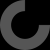MakerBot Print is our newest print-prepration software, which supports native CAD files and STL assemblies,
allows you to interact with all your printers via the Cloud, and many other exciting new features.

Download Now

# Report as inappropriate

the D in LED stands for diode. and a diode has an exponential function regarding current depending on voltage. once you are above the threshold voltage a small increase in voltage. (depending on the led: e.g 0.4v might double the current). thus it is very hard to predict the exact current when you apply a fixed voltage. get a little to high and you will exceed the allowed current (typically 20mA) and damage your led. so you need either ca current source (constant current) but typically you limit the current with a resistor.
alos having LEDs in parallel is not ideal: a small variation in their characteristic will lead to different currents flowing through them and thus different brightness. so an example: lets say you have a 9V battery and want a 3.2V white LED with 15mA. so you put a resistor in front of the LED: 9V-3.2V is 5.8V and to get 15mA you will 5.8V/15mA = 0.387kOhm. or 380Ohm. so you would e.g. choose 390Ohm.

Now small changes in the characteristic of the LED will still result in a different current but with the resistor it is no longer a problem. e.g. if the LED only need 3.1V instead of 3.2V the current would only increase by 0.1/5.8*100% or 1.7%. In the case where you would apply a fixed 3.2V the current might have incerase 300%.

now the downside of large resitors is: you are wasting energy there. in the exmaple above the LED gets 3.2v15mA which is 48mW where your restistor eats 5.8V15mA which is 87mW. A compromise would be to put LEDs in seriers here.

2 LEDs in series would mean 2*3.2V is 6.4V so for the resitor the voltage would be 9V-6.4V = 2.6V. at 15mA this would be: 0.173kOhm or 172Ohm so you would use: 180Ohm there. so you get the same brightness of the LEDs but only wasting 40%

with 3 white LEDs in seriers you would be on 9.6V. so the voltage is below the threshold voltage and the current would be rather small.

if you do not want to waste power and still guerantee a constant current then you need a more complicated curicuit that pulses the LEDs (and stores engery in an inductor).